# Innovation: Accuracy versus Precision

May 1, 2010  -#### A Primer on GPS Truth

By David Rutledge

True to its word origins, accuracy demands careful and thoughtful work. This article provides a close look at the differences between the precision and accuracy of GPS-determined positions, and should alleviate the confusion between the terms — making abuse of the truth perhaps less likely in the business of GPS positioning.

JACQUES-BÉNIGNE BOSSUET, the 17th century French bishop and pulpit orator, once said “Every error is truth abused.” He was referring to man’s foibles, of course, but this statement is much more general and equally well applies to measurements of all kinds. As I am fond of telling the students in my introduction to adjustment calculus course, there is no such thing as a perfect measurement. All measurements contain errors. To extract the most useful amount of information from the measurements, the errors must be properly analyzed.

Errors can be broadly grouped into two major categories: biases, which are systematic and which can be modeled in an equation describing the measurements, thereby removing or significantly reducing their effect; and noise or random error, each value of which cannot be modeled but whose statistical properties can be used to optimize the analysis results.

Take GPS carrier-phase measurements, for example. It is a standard approach to collect measurements at a reference station and a target station and to form the double differences of the measurements between pairs of satellites and the pair of receivers. By so doing, the biases in the modeled measurements that are common to both receivers, such as residual satellite clock error, are canceled or significantly reduced. However, the random error in the measurements due to receiver thermal noise and the quasi-random effect of multipath cannot be differenced away. If we estimate the coordinates of the target receiver at each epoch of the measurements, how far will they be from the true coordinates?

That depends on how well the biases were removed and the effects of random error. By comparing the results from many epochs of data, we might see that the coordinate values agree amongst themselves quite closely; they have high precision. But, due to some remaining bias, they are offset from the true value; their accuracy is low. Two different but complementary measures for assessing the quality of the results.

In this month’s column, we will examine the differences between the precision and accuracy of GPS-determined positions and, armed with a better understanding of these often confused terms, perhaps be less likely to abuse the truth in the business of GPS positioning.

“Innovation” features discussions about advances in GPS technology, its applications, and the fundamentals of GPS positioning. The column is coordinated by Richard Langley, Department of Geodesy and Geomatics Engineering, University of New Brunswick.

For many, Global Positioning System (GPS) measurement errors are a mystery. The standard literature rarely does justice to the complexity of the subject. A basic premise of this article is that despite this, most practical techniques to evaluate differential GPS measurement errors can be learned without great difficulty, and without the use of advanced mathematics. Modern statistics, a basic signal-processing framework, and the careful use of language allow these disruptive errors to be easily measured, categorized, and discussed.

The tools that we use today were developed over the last 350 years as mathematicians struggled to combine measurements and to quantify error, and to generally understand the natural patterns. A distinguished group of scientists carried out this work, including Adrien-Marie Legendre, Abraham de Moivre, and Carl Friedrich Gauss. These luminaries developed potent techniques to answer numerous and difficult questions about measurements.

We use two special terms to describe systems and methods that measure or estimate error. These terms are precision and accuracy. They are terms used to describe the relationship between measurements, and to underlying truth. Unfortunately, these two terms are often used loosely (or worse used interchangeably), in spite of their specific definitions. Adding to the confusion, accuracy is only properly understood when divided into its two natural components: internal accuracy and external accuracy.

GPS measurements are like many other signals in that with enough samples the probability distribution for each of the three components is typically bell-shaped, allowing us to use a particularly powerful error model. This bell-shaped distribution is often called a Gaussian distribution (after Carl Friedrich Gauss, the great German mathematician) or a normal distribution. Once enough GPS signal is accumulated, a normal distribution forms. Then, potent tools like Gauss’s normal curve error model and the associated square-root law can be brought to bear to estimate the measurement error.

An interesting aspect of GPS, however, is that over short periods of time, data are not normally distributed. This is of great importance because many applications are based upon small datasets. This results in a fundamental division in terms of how measurement error is evaluated. For short periods of time, the gain from averaging is difficult to quantify, and it may or may not improve accuracy. For longer periods of time the gain from averaging is significant, a normal distribution forms, and the square-root law is used to estimate the gain. The absence of a Gaussian distribution in these datasets (1 hour or less) is one source of the confusion surrounding measurement error. Another source of confusion is the richly nuanced concept of accuracy. By closely looking at each of these, a clear picture emerges about how to effectively analyze and describe differential GPS measurement error.

### The GPS Signal

It is helpful to consider consecutive differential GPS measurements as a signal, and thus from the vantage of signal processing. Here, we use the term measurement to refer to position solutions rather than the raw carrier-phase and pseudorange measurements a receiver makes. Sequential position measurements from a GPS system are discrete signals, the result of quantization, transformation, and other processing of the code and carrier data into more meaningful digital output. In comparison, a continuous signal is usually analog based and assumes a continuous range of values, like a DC voltage. A signal is a way of describing how one value is related to another.

Figure 1 shows a time series consisting of a discrete signal from a typical GPS dataset (height component). These data are based on processing carrier-phase data from a pair of GPS receivers, in double-difference mode, holding the position of one fixed while estimating that of the other. The vertical axis is often called the dependent variable and can be assigned many labels. Here it is labeled GPS height. The horizontal axis is typically called the independent variable, or the domain. This axis could be labeled either time or sample number, depending on how we want this variable to be represented. Here it is labeled sample number. The data in Figure 1 are in the time domain because each GPS measurement was sampled at equal intervals of time (1 second). We’ll refer to a particular data value (height) as xi.

Ten minutes of GPS data are displayed in Figure 1. These data are the first 600 measurements from a larger 96-hour dataset that forms the basis of this paper. The mean (or average) is the first number to calculate in any error-assessment work. The mean is indicated by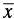. There is nothing fancy in computing the mean; simply add all of the measurements together and divide by the total sample number, or N. Equation 1 is its mathematical form:

The mean for these data is 474.2927 meters, and gives us the average value or “center” of the signal. By itself, the mean provides no information on the overall measurement error, so we start our investigation by calculating how far each GPS height determination is located away from the mean, or how the measurements spread or disperse away from the center. In mathematical form, the expression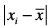denotes how far the ith sample differs from the mean.

As an example, the first sample deviates by 0.0038 meters (note that we always take the absolute value). The average deviation (or average error) is found by simply summing the deviations of all of the samples and dividing by N. The average deviation quantifies the spreading of the data away from the mean, and is a way of calculating precision. When the average deviation is small, we say the data are precise. For these data, the average deviation is 0.0044 meters.

For most GPS error studies, however, the average deviation is not used. Instead, we use the standard deviation where the averaging is done with power rather than amplitude. Each deviation from the mean,, is squared,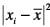, before taking the average. Then the square root is taken to adjust for the initial squaring. Equation 2 is the mathematical form of the standard deviation (SD):

The standard deviation for the data in Figure 1 is 0.0052 meters.

But note that these data have a changing mean (as indicated by the slowly varying trend). The statistical or random noise remains fairly constant, while the mean varies with time. Signals that change in this manner are called nonstationary. In this 10-minute dataset, the changing mean interferes with the calculation of the standard deviation. The standard deviation of this dataset is inflated to 0.0052 meters by the shifting mean, whereas if we broke the signal into one-minute pieces to compensate, it would be only 0.0026 meters.

To highlight this, Figure 2 is presented as an artificially created (or synthetic) dataset with a stationary mean equal to the first data point in Figure 1, and with the standard deviation set to 0.0026 meters. This figure, with its stable mean and consistent random noise, displays a Gaussian distribution (as we will soon see graphically), and illustrates what our dataset is not.

Contrasting these two datasets helps us to understand a critical aspect of differential GPS data. Analyzing a one-minute segment of GPS data from Figure 1 would provide a correct estimate of the standard deviation of the higher frequency random component, but would likely provide an incorrect estimate of the mean. This is because of its wandering nature; a priori we do not know which of the 10 one-minute segments is closer to the truth. It is tempting then to think that by calculating the statistics on the full 10 minutes we will conclusively have a better estimate of the mean, but this is not true.

The mean might be moving toward or away from truth over the time period. It is not yet centered over any one value because its distribution is not Gaussian. What’s more, when we calculate the statistics on the full 10 minutes of data, we will distort the standard deviation of the higher frequency random component upwards (from 0.0026 meters to 0.0052 meters).

This situation results in a great deal of confusion with respect to the study of GPS measurement error. When we look at Figures 1 and 2 side by side we see the complication. Figure 2 is a straightforward signal with stationary mean and Gaussian noise. Averaging a consecutive series of data points will improve the accuracy. Figure 1 is composed of a higher frequency random component (shown by the circle), plus a lower frequency non-random component. It is the superimposition of these two that causes the trouble. We cannot reliably calculate the increase in accuracy as we accumulate more data until the non-random component converges to a random process. This results in a very interesting situation; in numerous cases gathering more data can actually move the location parameter (the mean,) away from truth rather than toward it.

To fully understand the implications of this, consider its effect on estimating accuracy. If the mean is stationary, statistical methods developed by Gauss and others could be used to estimate the measurement error of an average for any set of N samples. For example, the so-called standard error of the average (SE) can be computed by taking the square root of the sample number, multiplying it by the standard deviation, and then dividing by the sample number (a method to provide an estimate of the error for any average that is randomly distributed). Equation 3 is its mathematical form: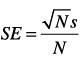                     

which simplifies to S/√N . This model can only be used if the data have a Gaussian distribution. Clearly this model cannot be used for the data in Figure 1, but can be used for the data in Figure 2. The implications are significant. The data from Figure 1 are not Gaussian because of the nonstationary mean, so we do not know if the gain from 10 minutes of averaging is better or worse than the first measurement. By contrast, the data in Figure 2 are Gaussian, so we know that the average of the series is more accurate than any individual measurement by a factor equal to the square root of the measurements.

By shifting these data into another domain we can see this more clearly. Figure 3 shows the 10 minutes of GPS data from Figure 1 plotted as a histogram or distribution of the number of data values falling within particular ranges of values. We call each range a bin. The histogram shows the frequencies with which given ranges of values occur. Hence it is also known as a frequency distribution. The frequency distribution can be converted to a probability distribution by dividing the bin totals by the total number of data values to give the relative frequency. If the number of observations is increased indefinitely and simultaneously the bin size is made smaller and smaller, the histogram will tend to a smooth continuous curve called a probability distribution or, more technically, a probability density function. A normal probability distribution curve is overlain in Figure 3 for perspective. This curve simultaneously demonstrates what a normal distribution looks like, and serves to graphically display the underlying truth (by showing the correct frequency distribution, mean, and standard deviation). It was generated by calculating the statistics of the 96-hour dataset, then using a random-number generator with adjustable mean and standard deviation (this is an example of internal accuracy, and will be discussed at length in an upcoming section). We can see that our Figure 1 dataset is not Gaussian because it does not have a credible bell shape. By contrast, when we convert the synthetic data from Figure 2 into a frequency distribution, we see the effect of the stationary mean — the data are distributed in a normal fashion because the mean is not wandering.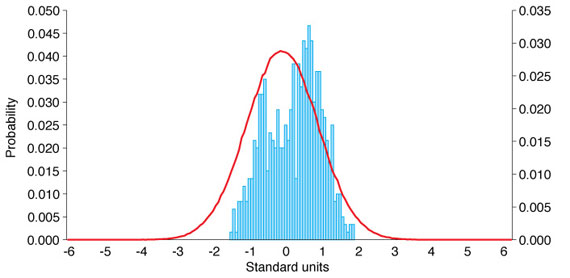Figure 3. Frequency distribution of a 10-minute sample of GPS height data.

Recall that all that is needed to use the Gauss model of measurement error is the presence of a random process. Mathematically, the measurement accuracy for the average of the data in Figures 1 and 3 is the overall standard deviation, or 0.0052 meters, because there is no gain per the square-root law. In comparison, the measurement accuracy for the average in Figure 4 is SE = (√ 600•0.0026) / 600 = 0.0001 meters. The standard deviation from the mean is still 0.0026 meters, but the accuracy of the averaged 600 samples is 0.0001 meters. Recall that precision is the spreading away from the mean, whereas accuracy is closeness to truth. When a process is normally distributed, the more data we collect the closer we come to underlying truth. The difference between the two is remarkable. Measurement error can be quickly beaten down when the frequency distribution is normal. This has significant implications for people who collect more than an hour of data, and raises the following question: At what point can we use the standard error model?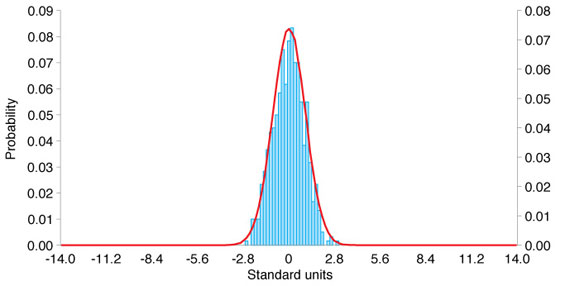Figure 4. Frequency distribution of a 10-minute sample of synthetic data.

### Frequency Distribution

In an ideal world, GPS data would display a Gaussian distribution over both short and long time intervals. This is not the case because of the combination of frequencies that we saw earlier (random + non-random). As an aside, this combination is a good example of why power is used rather than amplitude to calculate the deviation from the mean. When two signals combine, the resultant noise is equal to the combined power, and not amplitude.

Interesting things happen as we accumulate more data and continue our analysis of the 96-hour dataset. Earlier we discussed calculating the SD and the mean, and we looked at short intervals of GPS data in the time domain and the frequency-distribution domain. Moving forward, we will continue to look at the data in the frequency-distribution domain because it is far easier to recognize a Gaussian distribution there. The goal is to discover the approximate point at which GPS data behave in a Gaussian fashion as revealed by the appearance of a true bell curve distribution.

Figure 5 shows one minute of GPS data along with the “truth” curve for perspective. This normal curve, as discussed above, was generated using a random number generator with programmable SD and mean variables. The left axis shows the probability distribution for the GPS data, and the right axis shows the probability distribution function for the normal curve. This figure reinforces what we already know: one minute of GPS data are typically not Gaussian (Figure 3 shows the same thing for 10 minutes of data).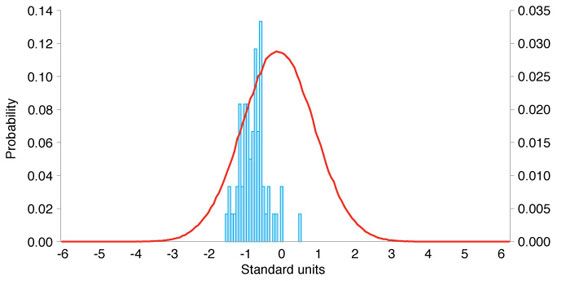Figure 5. Frequency distribution of a 1-minute sample of GPS height data.

Figure 6 shows 1 hour of GPS data. The data in Figure 6 show the beginnings of a clear normal distribution. Note that the mean of the GPS data is still shifted from the mean of the overall dataset. The appearance of a normal distribution at around 1 hour of data indicates that we can begin use of the standard error model, or the Gaussian error model. Recall that this states that the average of the collection of measurements is more accurate that any individual measurement by a factor equal to the square root of the number of measurements, provided the data follow the Gauss model and are normally distributed. For one hour of data, the gain is square root of 1 times the SD divided by N. In effect, no gain. But from this point forward each hour of data provides √N gain. Figure 7 shows 12 hours of data with a gain of √12. By calculating the standard error for the average of 12 hours of data, SE = (√12•0.0069)/12, or 0.0020 meters, we see a clear gain in accuracy. Notice also that at 12 hours the normal curve and the GPS data are close to being one and the same.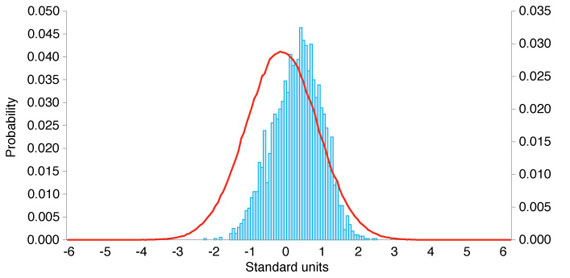Figure 6. Frequency distribution of a 1-hour sample of GPS height data.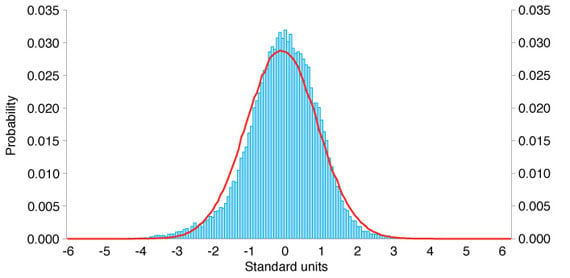Figure 7. Frequency distribution of a 12-hour sample of GPS height data.

Several things are worth pointing out here. The non-stationary mean converts to a Gaussian process after approximately 1 hour. There is nothing magical about this; conversion at some point is a necessary condition for the system to successfully operate. If it did not, the continually wandering mean would render it of little use as a commercial positioning system. Because it is non-stationary over the shorter occupations considered normal for many applications, it is confusing. Collecting more data in some instances can contribute to less accuracy. This situation also creates a gulf between those who collect an hour or two, and those who collect continuously. It is worth emphasizing that the distribution of data under our “truth” curve fills out nicely after 12 hours. This coincides with one pass of the GPS constellation, suggesting (as we already know) that a significant fraction of the wandering mean is affected by the geometrical error between the observer and the space vehicles overhead.

By looking at the 12 one-hour Gaussian distributions that comprise a 12-hour dataset, we see clearly what Francis Galton discovered in the 1800s. A normal mixture of normal distributions is itself normal, as Figure 8 shows. This sounds simple, but in fact it has significant implications. The unity between consecutive 1-hour segments of our dataset is the normal outline, reinforcing the increasing accuracy of the location parameter,, as more and more normal curves are summed together.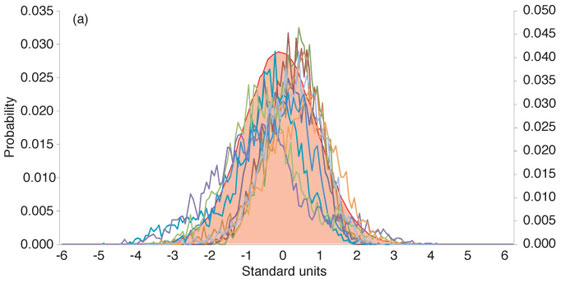######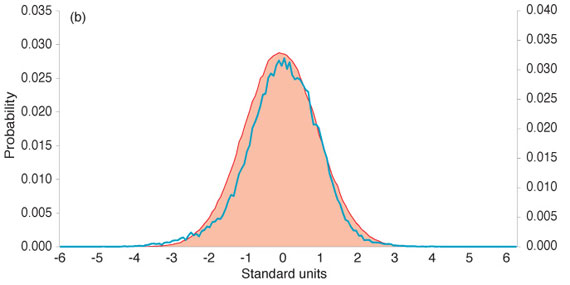Figure 8. (a) Frequency distribution of 12 1-hour samples of GPS height data; (b) the 12 1-hour samples combined.

### Internal vs. External Accuracy

Figure 9 shows the relationship between precision and accuracy. The dashed vertical line indicates the mean of the dataset (the inflection point at which the histogram balances). The red arrows bracket the spread of the dataset at 1 standard deviation from the mean (precision), while the black arrows bracket the offset of the mean from truth (accuracy). Notice that the mean () is a location parameter, while the standard deviation (<e
m>s) is a spread parameter. What we do with the mean is accuracy related; what we do with the standard deviation is precision related.

Accuracy is the difference between the true value and our best estimate of it. While the definition may be clear, the practice is not. Earlier we discussed two techniques used to calculate precision — the average deviation, and the standard deviation. We also discussed the square-root law that estimates the measurement error of a series of random measurements. As we saw, it was not possible to calculate this until roughly 1 hour of data had been collected. Furthermore, the data were said to be accurate when a good correlation appeared between the overlain curve and the GPS data at 12 hours.

But here is the interesting thing; the truth curve was derived internally. As previously discussed, data were accumulated for 96 hours, and then statistics were calculated on the overall dataset. Then a random number generator with programmable mean and standard deviation was used to generate a perfectly random distribution curve with the same location parameter and spread. This was declared as truth, and then smaller subsets of the same dataset were essentially compared with a perfect version of itself! This is an example of what is called internal accuracy.

By contrast, external accuracy is when a standard, another instrument, or some other reference system is brought to bear to gauge accuracy. A simple example is when a physical standard is used to confirm a length measurement. For instance, a laser measurement of 1 meter might be checked or calibrated against a 1-meter platinum iridium bar that is accepted as a standard. The important point here is that truth does not just appear — it has to be established through an internal or external process.

Accuracy can be evaluated in two ways: by using information internal to the data, and by using information external to the data. The historical development of measurement error is mostly about internal accuracy. Suppose that a set of astronomical measurements is subjected to mathematical analysis, without explicit reference to underlying truth. This is internal accuracy, and was famously expressed by Isaac Newton in Book Three of his Principia: “For all of this it is plain that these observations agree with theory, so far as they agree with one another.”

Internal accuracy constrains and simplifies the problem. It eliminates the need to bring other instruments or systems to bear. It makes the problem manageable by allowing us to use what we already have. Most importantly, it eliminates the need to consider point of view. Because we are not venturing outside of the dataset, it becomes the reference frame. By contrast, when you ponder bringing an external source of accuracy to bear it gets complicated, especially with GPS.

For example, is it sufficient to use one GPS receiver to check the accuracy of another, or should an entirely different instrument be used? Is it suitable to use the Earth-centered, Earth-fixed GPS frame to check itself, or should another frame be used? If we use another frame, should it extend beyond the Earth, or is it sufficient to consider accuracy from an Earth perspective? When we say a GPS measurement is accurate, what we are really saying is that it is accurate with respect to our reference frame. But what if you were an observer located on the Sun? An Earth-centric frame no longer makes sense when the point that you wish to measure is located on a planet that is rotating in an orbit around you. For an observer on the Sun, a Sun-centered, Sun-fixed reference frame would probably make more sense, and would result in easier to understand measurements. But we are not on the Sun, so a reference frame that rotates with the Earth — making fixed points appear static — makes the most sense. The difference between the two is that of perspective, and it can color our perception of accuracy.

Internal accuracy assessments sidestep these complications, but make it difficult to detect systematic errors or biases. Keep in mind that any given GPS measurement can be represented by the following equation: measurement = exact value + bias + random error. The random-error component presents roughly the same problem for both internal and external assessments. The bias however, requires external truth for detection. There is no easy way to detect a constant shift from truth in a dataset by studying only the shifted dataset.

In practice, people generally look for internal consistency, as Newton did. We look for consistency within a continuous dataset, or we collect multiple datasets at different times and then look for consistency between datasets. It is not uncommon to use the method taken in this article: let data accumulate until one is confident that the mean has revealed truth, and then use this for all further analysis. For this approach, accuracy implies how the measurements mathematically “agree with one another.”

All of this shows that accuracy is a very malleable term. Internal accuracy assumes that the process is centered over truth. It is implicitly understood that more measurements will increase the accuracy once the distribution is normal. The standard error is calculated by taking the square root of the sample number, multiplying it by the standard deviation, and then dividing by the sample number. With more samples, the standard error of the average decreases, and we say that the accuracy is increasing. Internal accuracy is a function of the standard deviation and the frequency distribution.

External accuracy derives truth from a source outside the dataset. Accuracy is the offset between this truth and the measurement, and not a function of the standard deviation of the dataset. The concept is simple, but in practice establishing an external standard for GPS can be quite challenging. For counterpoint, consider the convenient relationship between a carpenter and a tape measure. He is in the privileged position of carrying a replica of the truth standard. GPS users have no such tool. It is impossible to bring a surrogate of the GPS system to bear to check a measurement. Fortunately, new global navigation satellite systems are coming on line to help, but a formal analysis of how to externally check GPS accuracy leads one into a morass of difficult questions.

Accuracy is not a fundamental characteristic of a dataset like precision. This is why accuracy lacks a formal mathematical symbol. One needs to look no further than internal accuracy for the proof. For a dataset that is shifted away from truth, or biased, no amount of averaging will improve its accuracy. Because it is possible to be unaware of a bias using internal accuracy assessments, it follows that accuracy cannot be inherent to a dataset.

Looking at the interplay between mathematical notation and language provides more insight. For example, we describe the mathematical symbolwith the word mean. We don’t stop there, however; we also sometimes call it the average. Likewise, the mathematical symbol s is described by the words standard deviation, but we also know s as precision, sigma, repeatability, and sometimes spread. English has a wealth of synonyms, giving it an ability to describe that is unparalleled. In fact, it is one of only a few languages that require a thesaurus. This is why it is important to make a clear distinction between the relatively clear world of mathematical notation and the more free-form world of words. Language gives us flexibility and power, but can also confound with its ability to provide subtle differences in meaning.

When we look at the etymology of the word accuracy, we can see that it is aptly named. It comes from the Latin word accuro, which means to take care of, to prepare with care, to trouble about, and to do painstakingly. Accuro is itself derived from the root cura, which means roughly the same thing and is familiar to us today in the form of the word curator. It is fitting language for a process that requires so much care.

When we discuss measurement error we seldom use mathematical symbols; we use language that is every bit as important as the symbols. The word error itself derives from the Latin erro, which means to wander, or to stray, and suitably describes the random tendency of measurements.

Whether we describe it with mathematics or language, error describes a fundamental pattern we see in nature; independent measurements tend to randomly wander around a mean. When the frequency distribution is normal, accuracy from the underlying truth occurs in multiples of √N. Error is the umbrella covering the other terms because it is the natural starting point for any discussion. Because of this, precision and accuracy are naturally subsumed under error, with accuracy further split into internal and external accuracy. By contemplating all of this, we expose the healthy tension between words and mathematical notation. Neither is perfect. Mathematics establishes natural patterns and provides excellent approximation tools, but is not readily available to everyone. Language opens the door to perspective and point of view, and invites questions in a way that mathematical notation does not.

### Final Notes

Making sense of GPS error requires that we take a close look at the intricacies of the GPS signal, with particular attention to the ramp up to a normal distribution. It also requires a good hard look at the language of error. Shifting the GPS data back and forth between the frequency-distribution and time domains nicely illustrates the complications imposed by a non-stationary mean. Datasets that are an hour or less in duration do not always increase in accuracy when the measurements are averaged. Averaging may provide a gain, but it is not a certainty. When the non-stationary mean converges to a Gaussian process after an hour or so, we begin to see what De Moivre discovered almost 275 hundred years ago: accuracy increases as the square root of the sample size.

The GPS system is so good that the division of accuracy into its proper internal and external accuracy components is shimmering beneath the surface for most users. It is rare that a set of GPS measurements has a persistent bias, so internal accuracy assessments are usually appropriate. This should not stop us from being careful with how we discuss accuracy, however. Some attempt should be made to distinguish between the two types, and neither should be used interchangeably with precision. What’s more, while accuracy is not something intrinsic to a dataset like precision, it is still much more than just a descriptive word. Accuracy is the hinge between the formal world of mathematics and point of view. Its derivation from N and s in internal assessments stands in stark contrast to the more perspective-driven derivation often found in external assessments. When carrying out internal assessments, we must be aware that we are assuming that the measurements are centered over truth. When carrying out external assessments, we must be mindful of what outside mechanism we are using to provide truth. True to its word origins, accuracy demands careful and thoughtful work.

David Rutledge is the director for infrastructure monitoring at Leica Geosystems in the Americas. He has been involved in the GPS industry since 1995, and has overseen numerous high-accuracy GPS projects around the world.

• Highly Readable Texts on Basic Statistics and Probability
The Drunkard’s Walk: How Randomness Rules Our Lives by L. Mlodinow, Pantheon Books, New York, 2008.

Noise by B. Kosko,Viking Penguin, New York, 2006.

• Basic Texts on Statistics and Probability Theory
A Practical Guide to Data Analysis for Physical Science Students by Louis Lyons, Cambridge University Press, Cambridge, U.K., 1991.

Principles of Statistics by M.G. Bulmer, Dover Publications, Inc., New York, 1979.

• Relevant GPS World Articles
“Stochastic Models for GPS Positioning: An Empirical Approach” by R.F. Leandro and M.C. Santos in GPS World, Vol. 18, No. 2, February 2007, pp. 50–56.

“GNSS Accuracy: Lies, Damn Lies, and Statistics” by F. van Diggelen in GPS World, Vol. 18, No. 1, January 2007, pp. 26–32.

“Dam Stability: Assessing the Performance of a GPS Monitoring System” by D.R. Rutledge, S.Z. Meyerholtz, N.E. Brown, and C.S. Baldwin in GPS World, Vol. 17, No. 10, October 2006, pp. 26–33.

“Standard Positioning Service: Handheld GPS Receiver Accuracy” by C. Tiberius in GPS World, Vol. 14, No. 2, February 2003, pp. 44–51.

The Stochastics of GPS Observables” by C. Tiberius, N. Jonkman, and F. Kenselaar in GPS World, Vol. 10, No. 2, February 1999, pp. 49–54.

The GPS Observables” by R.B. Langley in GPS World, Vol. 4, No 4, April 1993, pp. 52–59.

The Mathematics of GPS” by R.B. Langley in GPS World, Vol. 2, No. 7, July/August 1991, pp. 45–50.